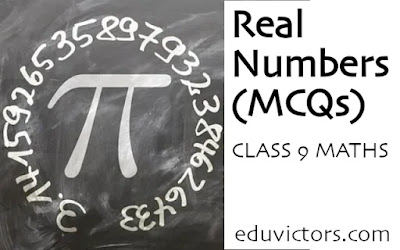# CBSE Class 9 - Maths - Real Numbers (MCQs)

Choose the correct option in each of the following questions:

Q1. Which of the following is a rational number?

(a) 2√3

(b) π

(c) 0

(d) √3 + 1

Q2. Every point on a number line represents

(a) a rational number

(b) a natural number

(c) an irrational number

(d) a real number

Q3: Every rational number is

(a) a natural number

(b) an integer

(c) a whole number

(d) a real number

Q4: The decimal representation of a rational number is

(a) always terminating

(b) either terminating or repeating

(c) either terminating or non-repeating

(d) neither terminating nor repeating

Q5: Product of (√3 + √2) and (√3 - √2) is

(a) a rational number

(b) an irrational number

(c) can be rational or irrational

(d) none of these

Q6: Between any two rational numbers there

(a) is no rational number

(b) is exactly one rational number

(c) are infinitely many rational numbers

(d) is no irrational number

Q7: Which of the following is an irrational number?

(a) 3.14

(b) 3.141414 ...

(c) 3.14444 ...

(d) 3.141141114 ...

Q8: Which of the following is a rational number?

(a) √2

(b) √9

(c) 11.010010001...

(d) π

Q9: The product of two irrational numbers is

(a) always irrational

(b) always rational

(c) always an integer

(d) sometimes rational and sometimes irrational

Q10: Decimal representation of 1/5 is:

(a) 0.2

(b) 0.5

(c) 0.02

(d) 0.002

1: (c) 0

2: (d) a real number

3: (d) a real number

4: (b) either terminating or repeating

5: (a) a rational number

6: (c) are infinitely many rational numbers

7: (d) 3.141141114 ...

8: (b) √9

9: (d) sometimes rational and sometimes irrational

10: (a) 0.2